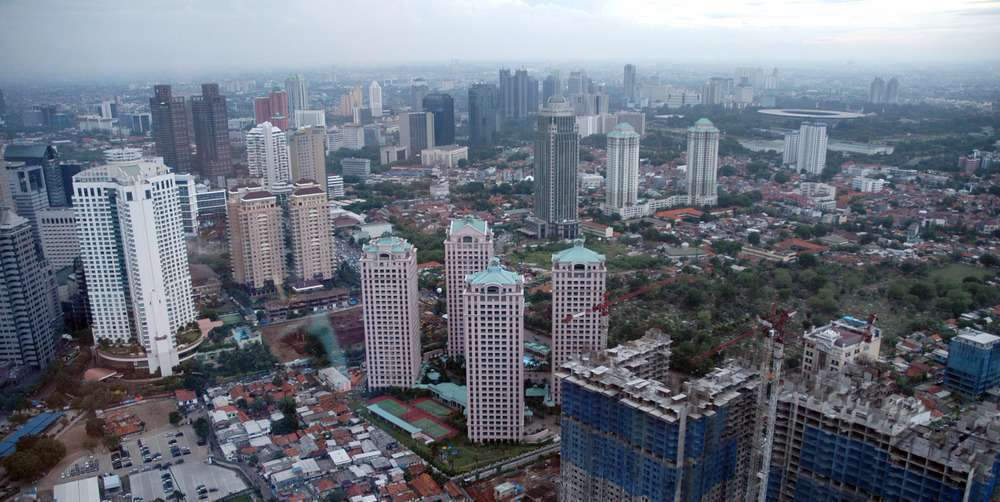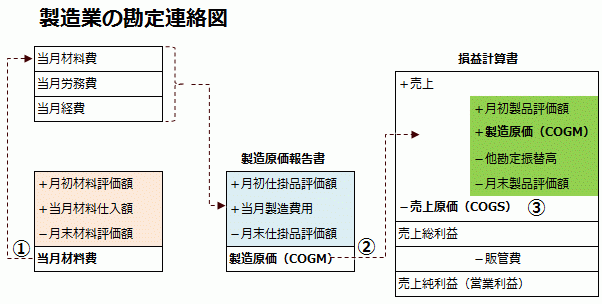# How to synchronize accounting and inventory in the system【Account Liaison Diagram】

2014/12/02## General account liaison diagram for manufacturing industry

In the chart of account liaison, on the statement of profit and loss (P/L), "sales - cost of sales" is gross profit, and cost of sales (COGS) is calculated by "products at the beginning of the month + manufacturing cost of the month - (transfer to other accounts + end-of-month products)".

The cost of goods manufactured during the month (COGS) is "products in progress at the beginning of the month + manufacturing cost of the month - products in progress at the end of the month," while manufacturing cost of the month consists of "material cost + manufacturing labor cost + manufacturing expenses".

Operating profit is gross profit minus SG&A expenses, and ordinary profit is ordinary profit after non-operating income and expenses are taken into account.## Periodic Method with Accounting Systems

In the periodic method asset account, the amount carried forward from the beginning of the month is transferred to a cost contra account called "opening stock" and the inventory balance at the end of the month is transferred to a cost contra account called "closing stock" based on the physical inventory.

These are the methods to determine the cost of sales in the manufacturing cost account together with the purchase, manufacturing cost (FOH) and labor cost in the periodic method journalization.## Periodic Method with Inventory Control System

At the end of the month, the system determines the valuation amount by the inventory balance update process, calculates the total average unit price in batches at the end of the month, determines the valuation amount by the inventory balance update process, and interfaces the inventory valuation amount with the accounting system.

This system is used in ERP system for non-manufacturing industry, but it is difficult to calculate the unit cost of inventory valuation, which takes into account the purchase cost of the purchased items and other factors, in the system.

## Periodic Method by cost management system

Materials and purchased parts are calculated on a total average unit cost basis.

• Total average unit price = (amount of inventory at the beginning of the month + actual purchases) / (amount of inventory at the beginning of the month + amount of purchases)

By multiplying the total average unit cost by the actual production volume, the direct material cost per item (work-in-process and product) is calculated.

For fixed costs such as labor costs and manufacturing overhead costs, the trial balance (T/B) is aggregated from indirect departments to cost centers (direct departments, product groups, etc.), and then the direct cost center section allocates the cost proportionally to the item.

Instead of calculating material consumption, manufacturing cost and sales cost inductively by the trichotomous method, the cost management system calculates the unit cost of material for the month by the gross average method and calculates the material consumption deductively.

On the other hand, fixed costs are added to the direct material cost of the product by dividing the amount of incurred costs inductively by the direct man-hours, and the difference is that COGS is calculated by multiplying the unit cost of the product by the actual sales volume.

If you want to allocate manufacturing overhead cost to a department and to know profit and loss by department in ledger (G/L), you need to divide the indirect labor cost account into two separate accounts, one for the molding department and one for the processing department, and make a re-class journal entry in the manual, but you need to enter the department code at the time of expense posting.

• Dr. Indirect Labor Cost　　10,000　　　　Cr. Deposit　　10,000

When performing a transfer journal entry based on the results of the allocation calculation in the cost management system

• Dr. Indirect Labor（Molding）　　40,000　　　　Cr. Indirect Labor　　40,000
• Dr. Indirect Labor（Processing）　　60,000　　　　Cr. Indirect Labor　　60,000

## Perpetual Method, a production management system

In the original actual costing, direct material cost is calculated from the total average unit cost calculated at the end of the month, and direct labor cost and indirect cost are allocated according to the working hours and volume of work by aggregating the expenses incurred in the month from G/L (General Ledger General Accounting).

The direct material cost unit price is updated by the moving average method as needed, and the standard unit price of the fixed cost is stored in the item master or BOM (bill of materials composition).

According to the input performance of material, material as asset account is transferred to material consumption as expense account, and material cost and fixed cost are transferred to work-in-process through contra account (relative account), and the receiving and paying journal of input and production is generated in real time, and the movement of inventory is reflected in accounting B/S.

Since the fixed cost is fixed at the end of the month, the product valuation is the "amount of materials used (moving average) + standard fixed cost," so at the end of the month, we need to make a journal entry to adjust the difference between the amount of actual fixed cost incurred and the amount of fixed cost.

Then, each time a shipment is made, the product is transferred to the cost of sales, so that inventory, P/L and B/S are synchronized with each other even during the month.

This is because we want to get the balance of material consumption at the end of the month from G/L. The reason why we use Material Consumption Contra Account instead of directly reducing the amount of material consumption from material, which is an asset account, is because we want to get the balance of material consumption from G/L at the end of the month.

1. The incoming inventory (materials) is actually recorded in the purchase input.

• Dr. R/M 10　　　　Cr. A/P 10

2.Materials are transferred to the material cost based on the input results. At this time, the unit price of material is the moving average price.

• Dr. Material Cost 10　　　　Cr. R/M 10

3.The current production cost is transferred from the actual production to the contra account. Until the actual cost is determined at the end of the month, the standard unit price set in the BOM is used for the fixed costs incurred in this process. In this case, the negative cost is posted to the contra account first.

• Dr. WIP 10　　　　Cr. Material Cost-Offset 10
• Dr. WIP 5　　　　Cr. FOH-Offset (Cost credit) 5
• Dr. WIP 30　　　　Cr. Labor Cost-Offset (Cost credit) 30

4.The amount of work-in-process is transferred to the manufacturing cost.

• Dr. COGM 45　　　　Cr. WIP 45

5.Re-classify (reclassify) the product with a manufacturing cost contra.

• Dr. FG 45　　　　Cr. COGM-Offset 45

6.Inventory (F/G) for the shipment is transferred to cost of sales upon sales registration.

• Dr. COGS 45　　　　Cr. F/G 45

7.The difference in fixed costs is divided proportionally between products (month-end inventory) and cost of sales.

• Dr. F/G 0.2　　　　Cr. FOH-Offset 1
• Dr. COGS 0.8